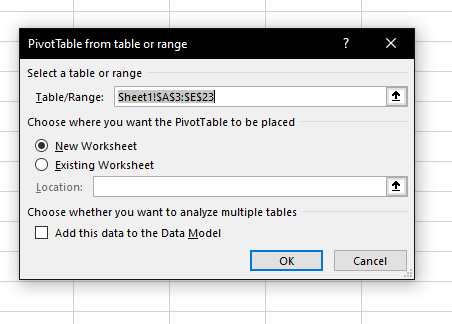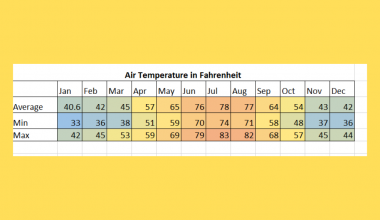# How to Make a General Ledger in Excel

This guide will explain how to set up a general ledger in Excel.

General ledgers are used to keep track of transactions. A general ledger is a commonly used record in accounting and contains both debit and credit transactions.

Ledgers typically contain all past transactions of a particular company or organization. Each transaction must include detailed information such as the date, amount, and description.

Let’s take a look at a quick example of what you might find in a general ledger.

Suppose you are an accountant for a bank. You want to keep track of the credit and debit transactions that occur daily.

Each transaction will have a unique transaction ID, a brief description, the date the transaction, and the amount associated with the transaction.

In Excel, we can create a table for our ledger. This table will contain all relevant information, each row being a single transaction.

After populating the table with transactions, we can create a pivot table to summarize our ledger. We may also add a Total row to our ledger so that the user can see the total in the same view.

Now that we have a grasp on when to use a general ledger, let’s take a look at an actual spreadsheet that uses a general ledger.

## A Real Example of a General Ledger in Excel

The following section provides several examples of how to use this function. We will also go into detail about the formulas and tools used in these examples.

First, let’s take a look at a real example of an Excel spreadsheet that has a functional general ledger.

In the example below, we have a general ledger template that has been filled with twenty transactions.To get the total count of transactions in the second column, we can use the following formula:

`=COUNT(B4:B23)`

To get the sum of all debit transactions, we can use this formula:

`=SUM(D4:D23)`

Do you want to take a closer look at our examples? You can make your own copy of the spreadsheet above using the link attached below.

Use our sample spreadsheet to add a test transaction to see how it changes the total. If you’re ready to try creating your own general ledger in Excel, head over to the next section and follow our guide on how to do it!

## How to Make a General Ledger in Excel

This section will guide you through each step needed to create your own general ledger in Microsoft Excel.

You’ll learn how to format a table to hold all the necessary information needed for each transaction. Learn how to use the ledger itself as a data source for an Excel PivotTable for additional analysis.

Follow these steps to set up a general ledger for accounting in Excel:

1. Create the initial template where you will place transactions. In this example, we’ll use a simple table with basic fields such as transaction ID, transaction date, and description. We have separate columns for debit and credit.2. In the example below, we’ve added sample transactions to our table.3. Next, we’ll modify the formatting for the Debit and Credit fields to follow the typical formatting for accounting ledgers. We can use the Accounting Number Format option in the Home tab.4. To create a Pivot Table using our ledger data, navigate to the Insert tab. Click on the PivotTable option and click From Table/Range in the dropdown menu.5. In the new dialog popup, you may choose whether to place the PivotTable in a new worksheet or in an existing worksheet. For this example, we’ll place the PivotTable in a new worksheet. Click on OK to proceed.6. We can adjust the PivotTable to track different fields from our ledger. For example, the PivotTable below divides each transaction by its description. Each category is further subdivided into the month the transaction took place.7. We can also add a Total row to our original general ledger. For the total in column B, we will use the `COUNT` function to give the number of transactions in the ledger. We’ll use the `SUM` function to get the final total for both credit and debit transactions.These are all the steps needed to make a basic general ledger in Microsoft Excel.

This detailed guide should provide you with all the information you need to create your own general ledger in Excel.

We’ve shown how to make a general ledger step-by-step. You should now also know how to use the PivotTable tool for further analysis of your transactions.

This function is just one example of the many Excel functions that you can use in your spreadsheets. Overall, our website offers hundreds of other functions and methods to help you get more out of Microsoft Excel.

With so many other Excel functions available, you can find one appropriate for your use case.

Don’t miss out on new spreadsheet tips, tricks, and best practices from our team. Subscribe to our newsletter to stay updated on the latest guides from us!### Get emails from us about Excel.

Our goal this year is to create lots of rich, bite-sized tutorials for Excel users like you. If you liked this one, you'd love what we are working on! Readers receive ✨ early access ✨ to new content.

##### You May Also Like## How to Make a Heat Map With or Without Numbers in Excel

This guide will show you how you can set up a heat map with or without numbers in…## How to Perform a VLOOKUP with Time Range in Excel

This guide will explain how you can use VLOOKUP with a given time range in Excel. We can…## How to Protect Sheet but Allow Data Entry in Excel

This guide will explain how you can protect a sheet but still allow data entry in Excel. Excel’s…## How to Calculate Cumulative Percentage in Excel

This guide will explain how you can calculate the cumulative percentage in Excel. In mathematics, the cumulative percentage…